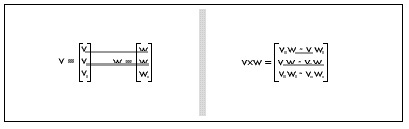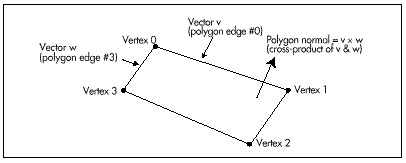For a single convex polyhedron, removal of polygons that aren’t facing the viewer would solve all hidden surface problems. In a convex polyhedron, any polygon facing the viewer can never be obscured by any other polygon in that polyhedron; this falls out of the definition of a convex polyhedron. Likewise, any polygon facing away from the viewer can never be visible. Therefore, in order to draw a convex polyhedron, if you draw all polygons facing toward the viewer but none facing away from the viewer, everything will work out properly, with no additional checking for overlap and hidden surfaces needed.

Unfortunately, backface removal completely solves the hidden surface problem for convex polyhedrons only, and only if there’s a single convex polyhedron involved; when convex polyhedrons overlap, other methods must be used. Nonetheless, backface removal does instantly halve the number of polygons to be handled in rendering any particular scene. Backface removal can also speed hidden-surface handling if objects are built out of convex polyhedrons. In this chapter, though, we have only one convex polyhedron to deal with, so backface removal alone will do the trick.

Given that I’ve convinced you that backface removal would be a handy thing to have, how do we actually do it? A logical approach, often implemented in the PC literature, would be to calculate the plane equation for the plane in which the polygon lies, and see which way the normal (perpendicular) vector to the plane points. That works, but there’s a more efficient way to calculate the normal to the polygon: as the cross-product of two of the polygon’s edges.

The cross-product of two vectors is defined as the vector shown in Figure 51.1. One interesting property of the cross-product vector is that it is perpendicular to the plane in which the two original vectors lie. If we take the cross-product of the vectors that form two edges of a polygon, the result will be a vector perpendicular to the polygon; then, we’ll know that the polygon is visible if and only if the cross-product vector points toward the viewer. We need one more thing to make the cross-product approach work, though. The cross-product can actually point either way, depending on which edges of the polygon we choose to work with and the order in which we evaluate them, so we must establish some conventions for defining polygons and evaluating the cross-product.Figure 51.1
The cross-product of two vectors.

We’ll define only convex polygons, with the vertices defined in clockwise order, as viewed from the outside; that is, if you’re looking at the visible side of the polygon, the vertices will appear in the polygon definition in clockwise order. With those assumptions, the cross-product becomes a quick and easy indicator of polygon orientation with respect to the viewer; we’ll calculate it as the cross-product of the first and last vectors in a polygon, as shown in Figure 51.2, and if it’s pointing toward the viewer, we’ll know that the polygon is visible. Actually, we don’t even have to calculate the entire cross-product vector, because the Z component alone suffices to tell us which way the polygon is facing: positive Z means visible, negative Z means not. The Z component can be calculated very efficiently, with only two multiplies and a subtraction.

The question remains of the proper space in which to perform backface removal. There’s a temptation to perform it in view space, which is, after all, the space defined with respect to the viewer, but view space is not a good choice. Screen space—the space in which perspective projection has been performed—is the best choice. The purpose of backface removal is to determine whether each polygon is visible to the viewer, and, despite its name, view space does not provide that information; unlike screen space, it does not reflect perspective effects.Figure 51.2
Using the cross product to generate a polygon normal.

Backface removal may also be performed using the polygon vertices in screen coordinates, which are integers. This is less accurate than using the screen space coordinates, which are floating point, but is, by the same token, faster. In Listing 51.3, which we’ll discuss shortly, backface removal is performed in screen coordinates in the interests of speed.

Backface removal, as implemented in Listing 51.3, will not work reliably if the polygon is not convex, if the vertices don’t appear in clockwise order, if either the first or last edge in a polygon has zero length, or if the first and last edges are collinear. These latter two points are the reason it’s preferable to work in screen space rather than screen coordinates (which suffer from rounding problems), speed considerations aside.

Backface Removal in Action

Listings 51.1 through 51.5 together form a program that rotates a solid cube in real-time under user control. Listing 51.1 is the main program; Listing 51.2 performs transformation and projection; Listing 51.3 performs backface removal and draws visible faces; Listing 51.4 concatenates incremental rotations to the object-to-world transformation matrix; Listing 51.5 is the general header file. Also required from previous chapters are: Listings 50.1 and 50.2 from Chapter 50 (draw clipped line list, matrix math functions); Listings 47.1 and 47.6 from Chapter 47, (Mode X mode set, rectangle fill); Listing 49.6 from Chapter 49; Listing 39.4 from Chapter 39 (polygon edge scan); and the FillConvexPolygon() function from Listing 38.1 from Chapter 38. All necessary modules, along with a project file, will be present in the subdirectory for this chapter on the listings diskette, whether they were presented in this chapter or some earlier chapter. This may crowd the listings diskette a little bit, but it will certainly reduce confusion!

Graphics Programming Black Book © 2001 Michael Abrash